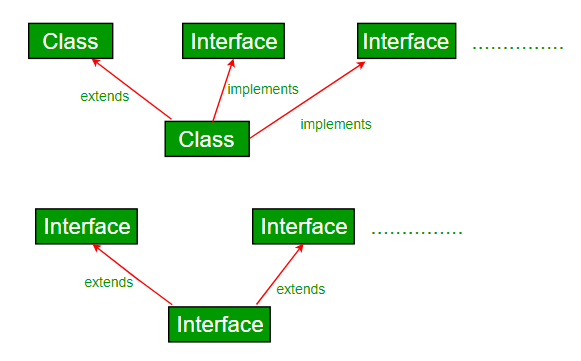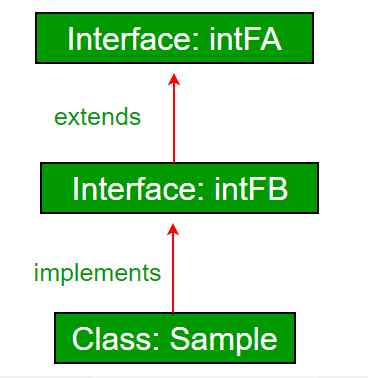# Interfaces and Inheritance in Java

• Difficulty Level : Easy
• Last Updated : 28 Jun, 2021

Prerequisites: Interfaces in Java, Java and Multiple Inheritance

A class can extends another class and/ can implement one and more than one interface.`// Java program to demonstrate that a class can``// implement multiple interfaces``import` `java.io.*;``interface` `intfA``{``    ``void` `m1();``}`` ` `interface` `intfB``{``    ``void` `m2();``}`` ` `// class implements both interfaces``// and provides implementation to the method.``class` `sample ``implements` `intfA, intfB``{``    ``@Override``    ``public` `void` `m1()``    ``{``        ``System.out.println(``"Welcome: inside the method m1"``);``    ``}`` ` `    ``@Override``    ``public` `void` `m2()``    ``{``        ``System.out.println(``"Welcome: inside the method m2"``);``    ``}``}`` ` `class` `GFG``{``    ``public` `static` `void` `main (String[] args)``    ``{``        ``sample ob1 = ``new` `sample();`` ` `        ``// calling the method implemented``        ``// within the class.``        ``ob1.m1();``        ``ob1.m2();``    ``}``}`

Output;

```Welcome: inside the method m1
Welcome: inside the method m2
```

Interface inheritance : An Interface can extend other interface.`// Java program to demonstrate inheritance in ``// interfaces.``import` `java.io.*;``interface` `intfA``{``    ``void` `geekName();``}`` ` `interface` `intfB ``extends` `intfA``{``    ``void` `geekInstitute();``}`` ` `// class implements both interfaces and provides``// implementation to the method.``class` `sample ``implements` `intfB``{``    ``@Override``    ``public` `void` `geekName()``    ``{``        ``System.out.println(``"Rohit"``);``    ``}`` ` `    ``@Override``    ``public` `void` `geekInstitute()``    ``{``        ``System.out.println(``"JIIT"``);``    ``}`` ` `    ``public` `static` `void` `main (String[] args)``    ``{``        ``sample ob1 = ``new` `sample();`` ` `        ``// calling the method implemented``        ``// within the class.``        ``ob1.geekName();``        ``ob1.geekInstitute();``    ``}``}`

Output:

```Rohit
JIIT
```

An interface can also extend multiple interfaces.

 `// Java program to demonstrate multiple inheritance ``// in interfaces``import` `java.io.*;``interface` `intfA``{``    ``void` `geekName();``}`` ` `interface` `intfB ``{``    ``void` `geekInstitute();``}`` ` `interface` `intfC ``extends` `intfA, intfB ``{``    ``void` `geekBranch();``}`` ` `// class implements both interfaces and provides``// implementation to the method.``class` `sample ``implements` `intfC``{``    ``public` `void` `geekName()``    ``{``        ``System.out.println(``"Rohit"``);``    ``}`` ` `    ``public` `void` `geekInstitute()``    ``{``        ``System.out.println(``"JIIT"``);``    ``}`` ` `    ``public` `void` `geekBranch()``    ``{``        ``System.out.println(``"CSE"``);``    ``}``     ` `    ``public` `static` `void` `main (String[] args)``    ``{``        ``sample ob1 = ``new` `sample();`` ` `        ``// calling the method implemented``        ``// within the class.``        ``ob1.geekName();``        ``ob1.geekInstitute();``        ``ob1.geekBranch();``    ``}``}`

Output:

```Rohit
JIIT
CSE
```

Why Multiple Inheritance is not supported through a class in Java, but it can be possible through the interface?
Multiple Inheritance is not supported by class because of ambiguity. In case of interface, there is no ambiguity because implementation to the method(s) is provided by the implementing class up to Java 7. From Java 8, interfaces also have implementations of methods. So if a class implementing two or more interfaces having the same method signature with implementation, it is mandated to implement the method in class also. Refer Java and Multiple Inheritance for details.

This article is contributed by Nitsdheerendra. If you like GeeksforGeeks and would like to contribute, you can also write an article using write.geeksforgeeks.org or mail your article to review-team@geeksforgeeks.org. See your article appearing on the GeeksforGeeks main page and help other Geeks.